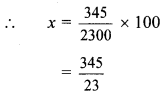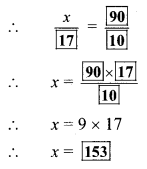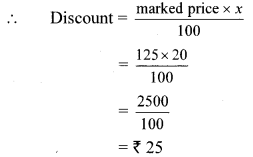# Maharashtra Board Class 8 Maths Solutions Chapter 9 Discount and Commission Practice Set 9.1

## Maharashtra State Board Class 8 Maths Solutions Chapter 9 Discount and Commission Practice Set 9.1

8th Standard Maths Practice Set 9.1 Question 1. If marked price = Rs 1700, selling price = Rs 1540, then find the discount.
Solution:
Here, Marked price = Rs 1700,
selling price = Rs 1540
Selling price = Marked price – Discount
∴ 1540 = 1700 – Discount
∴ Discount = 1700 – 1540
= Rs 160
∴ The amount of discount is Rs 160.

Discount and Commission Practice Set 9.1 Question 2. If marked price Rs 990 and percentage of discount is 10, then find the selling price.
Solution:
Here, marked price = Rs 990,
discount = 10%
Let the percentage of discount be x
∴ x = 10%
i. Discount= Rs 99

ii. Selling price = Marked price – Discount
= 990 – 99
= Rs 891
∴ The selling price is Rs 891.

Practice Set 9.1 Question 3. If selling price Rs 900, discount is 20%, then find the marked price.
Solution:
Here, selling price = Rs 900, discount = 20%
Let the marked price be Rs 100
Since, the discount given = 20%
∴ Amount of discount = Rs 20
∴ Selling price = 100 – 20 – Rs 80
Let actual marked price be Rs x
∴ For marked price of Rs x, selling price is Rs 900
$$\frac{80}{100}=\frac{900}{x}$$
∴ 80 × x = 100 × 900
∴ $$x=\frac{100 \times 900}{80}$$
∴ x = Rs 1125
∴ The marked price is Rs 1125.

Discount and Commission Std 8 Question 4. The marked price of the fan is Rs 3000. Shopkeeper gave 12% discount on it. Find the total discount and selling price of the fan.
Solution:
Here, Marked price = Rs 3000, discount = 12%
Let the percentage of discount be x.
∴ x = 12%
i. Discount= 30 × 12
= Rs 360

ii. Selling price = Marked price – Discount
= 3000 – 360
= Rs 2640
∴ The total discount is Rs 360 and the selling price of the fan is Rs 2640.

Discount and Commission 8th Standard Question 5. The marked price of a mixer is Rs 2300. A customer purchased it for Rs 1955. Find percentage of discount offered to the customer.
Solution:
Here, marked price = Rs 2300,
selling price = Rs 1955
i. Selling price = Marked price – Discount
∴ 1955 = 2300 – Discount
∴ Discount = 2300 – 1955
= Rs 345

ii. Let the percentage of discount be x∴ x = 15%
∴ The percentage of discount offered to the customer is 15%.

Question 6.
A shopkeeper gives 11% discount on a television set, hence the cost price of it is Rs 22,250. Then find the marked price of the television set.
Solution:
Here, selling price = Rs 22,250, discount = 11%
Let marked price be Rs 100
Since, the discount given = 11%
∴ Amount of discount = Rs 11
∴ Selling price = 100 – 11 = Rs 89
∴ Let actual marked price be Rs x
∴ For marked price of Rs x, selling price is Rs 22,250∴ x = Rs 25,000
∴ The marked price of the television set is Rs 25,000.

8th Std Maths Discount and Commission Question 7. After offering discount of 10% on marked price, a customer gets total discount of Rs 17. To find the cost price for the customer, fill in the following boxes with appropriate numbers and complete the activity.
Solution:
Suppose, marked price of the item = 100 rupees Therefore, for customer that item costs 100 – 10 = 90 rupees.
Hence, when the discount is  then the selling price is  rupees.
Suppose when the discount is  rupees, the selling price is x rupees.∴ The customer will get the item for Rs 153.

Question 8.
A shopkeeper decides to sell a certain item at a certain price. He tags the price on the item by increasing the decided price by 25%. While selling the item, he offers 20% discount. Find how many more or less percent he gets on the decided price.
Solution:
Here, price increase = 25%,
discount offered = 20%
Let the decided price be Rs 100
∴ Increase in price = Rs 25
∴ Shopkeeper marks the price = 100 + 25
= Rs 125
∴ marked price = Rs 125
Let the percentage of discount be x
∴ x = 20%∴ Selling price = Marked price – Discount
= 125 – 25
= Rs 100
∴ If the decided price is Rs 100, then shopkeeper gets Rs 100.
∴ The shopkeeper gets neither more nor less than the decided price i.e. he gets 0% more / less.

Maharashtra Board Class 8 Maths Chapter 9 Discount and Commission Practice Set 9.1 Intext Questions and Activities

Question 1.
Write the appropriate numbers in the following boxes. (Textbook pg. no. 51)

1. $$\frac { 12 }{ 100 }=$$ __ percent = __%
2. 47% = __
3. 86% = __
4. 4% of 300 = 300 × __ = __
5. 15% of 1700 = 1700 × __= __

Solution:

1. $$\frac { 12 }{ 100 }=$$ 12 percent = 12%
2. 47% = $$\frac { 47 }{ 100 }$$
3. 86% = $$\frac { 86 }{ 100 }$$
4. 4% of 300 = 300 × $$\frac { 4 }{ 100 }$$ = 12
5. 15% of 1700 = 1700 × $$\frac { 15 }{ 100 }$$ = 255

Question 2.
You may have seen advertisements like ‘Monsoon Sale’, ‘Stock Clearance Sale’ etc offering different discount. In such a sale, a discount is offered on various goods. Generally in the month of July, sales of clothes are declared. Find and discuss the purpose of such sales. (Textbook pg. no. 51)
Solution:
(Students should attempt the above activity on their own)